﻿ Backstep flow (Inflow rate mapping) - XSim

# Backstep flow (Inflow rate mapping)

Update: June 1, 2017
OpenFOAM 4.x

## Case directory

\$FOAM_TUTORIALS/incompressible/pisoFoam/les/pitzDailyMapped

## Summary

The fluid flows in from the region "inlet" and out from the region "outlet". No-slip condition is set to the walls of the flow channel, and the problem is solved as 2-dimensional problem with a single mesh in the Z-direction. LES (Eddy viscosity model is dynamic one-equation) is used for turbulence calculation.Model geometry

By setting a "mapped" type condition as the inflow condition, the inflow rate is set by referring to the value of the area offset by 0.0495 m in the X direction from the region "inlet". This setting is specified in the 0/U and constant/polyMesh/boundary files as follows.

```inlet
{
type                mapped;
value               uniform (10 0 0);
interpolationScheme cell;
setAverage          true;
average             (10 0 0);
}
```
File 0/U
```inlet
{
type                mapped;
value               uniform (10 0 0);
interpolationScheme cell;
setAverage          true;
average             (10 0 0);
}
```
File constant/polyMesh/boundary

The meshes are as follows.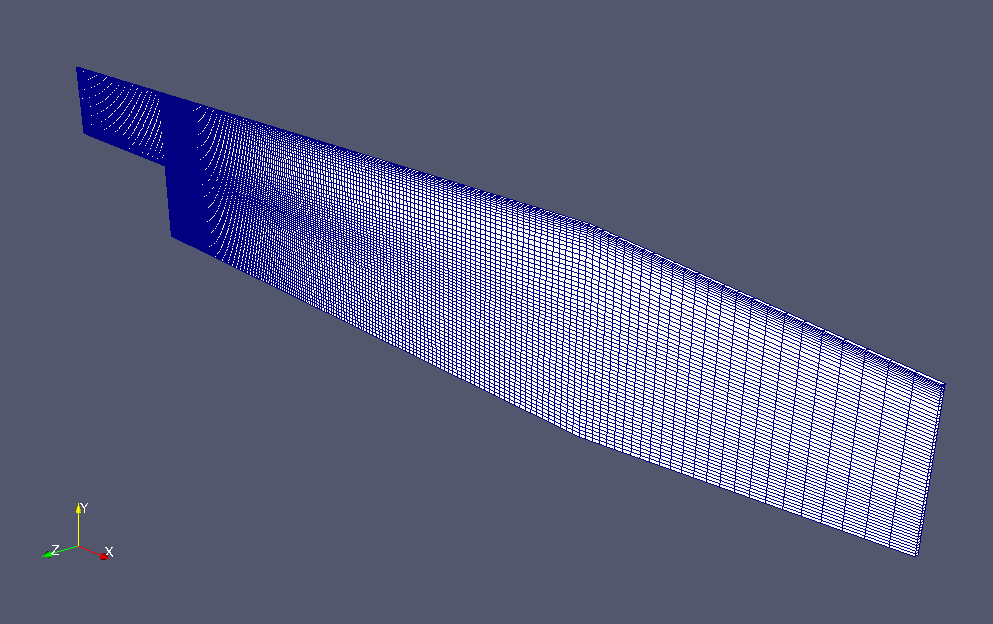Meshes

The pressure at each time at the specified coordinates is output using the probes function, and the flow velocity at each time on the specified surface is output using the surfaceSampling function, under the postProcessing directory. Using the fieldAverage function, the time averages of the pressure and velocity are output as pMean and UMean for each time. These settings are made in system/controlDict.

The calculation result is as follows.

Flow velocity (U)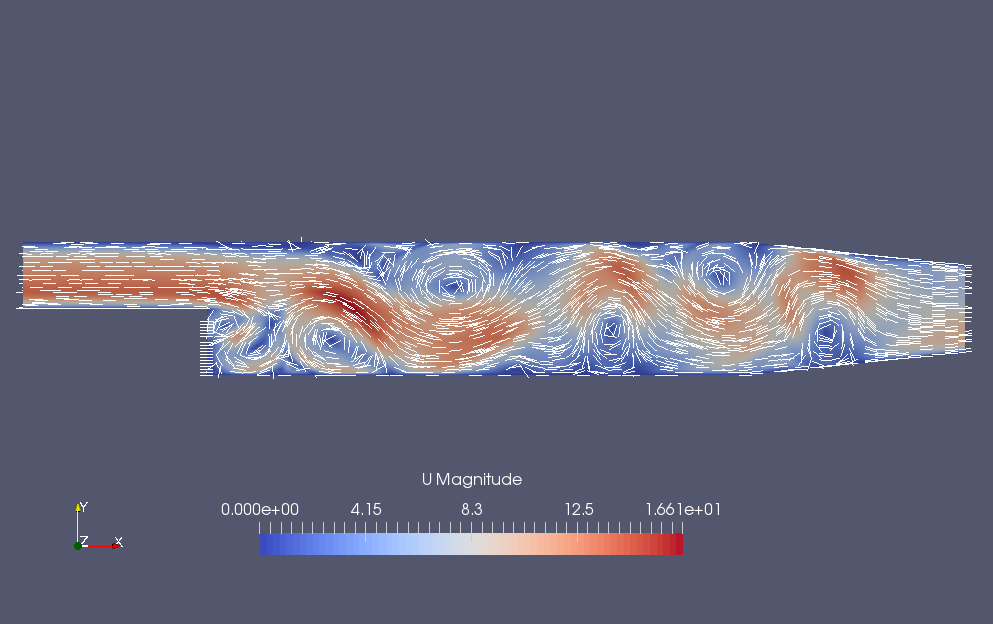Flow velocity (U)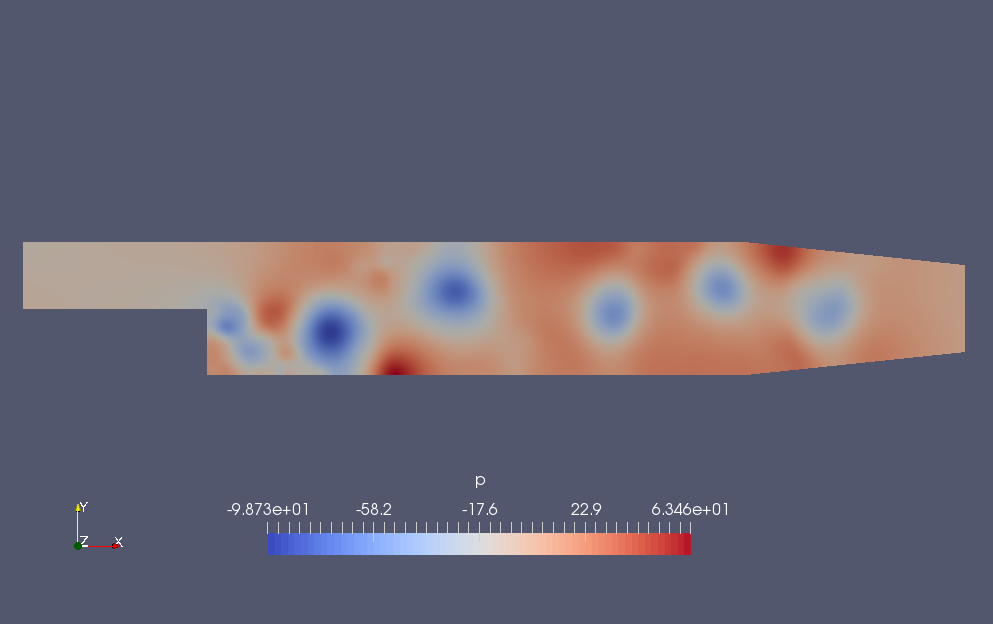Pressure (p)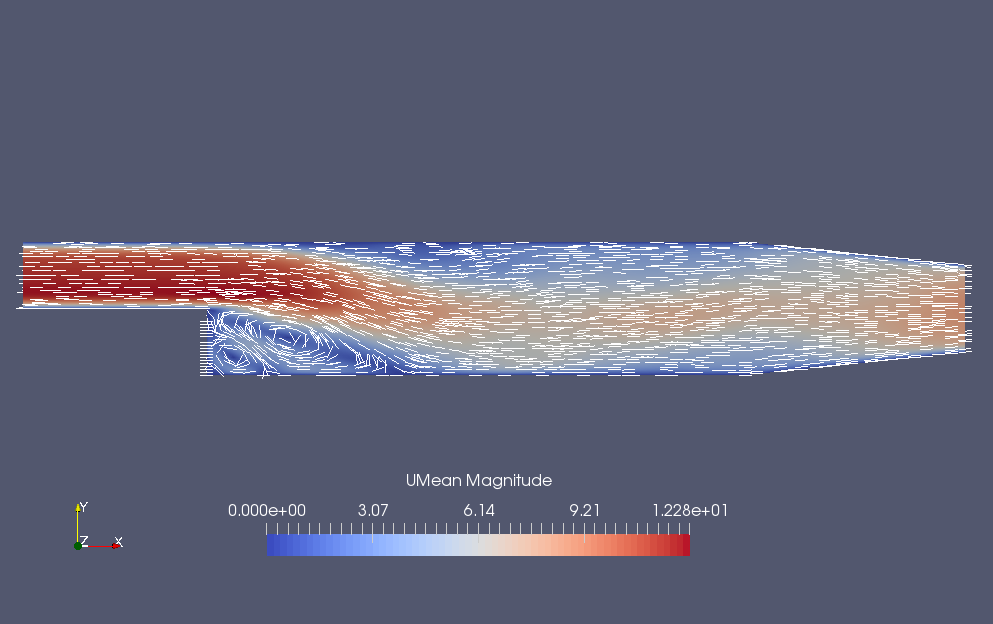Time-averaged flow velocity at the final time (UMean)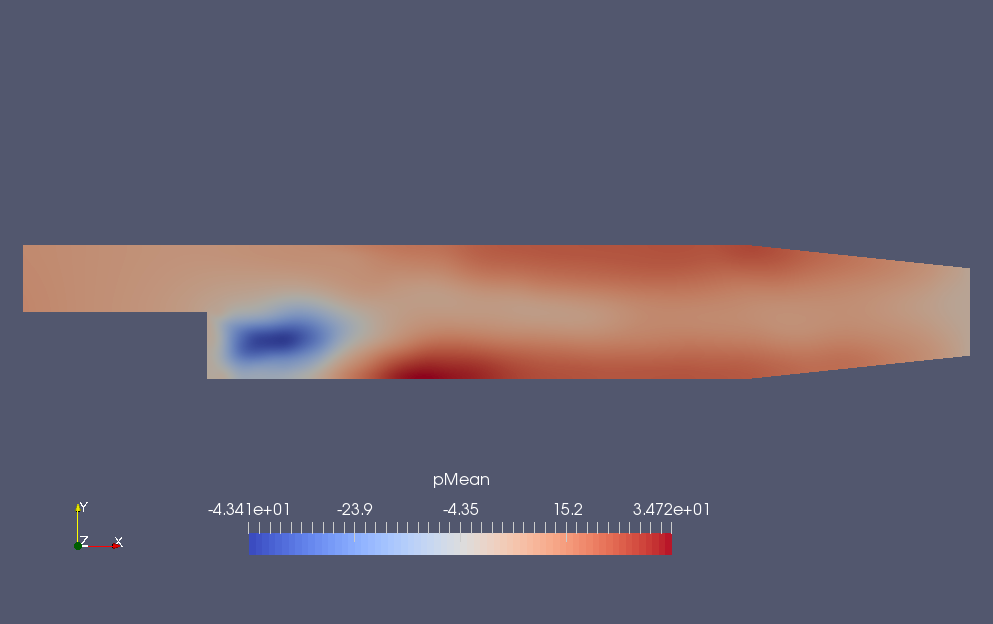Time-averaged pressure at the final time (pMean)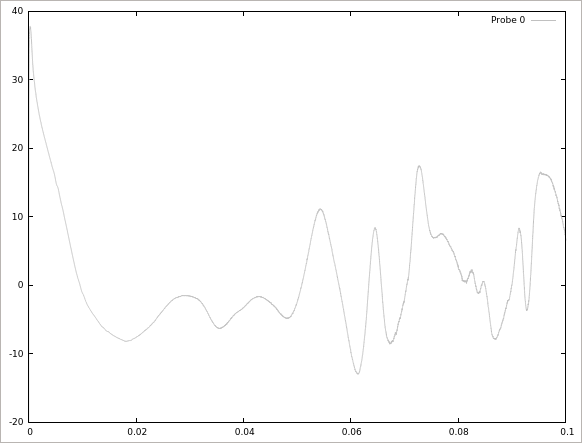Time history of pressure at coordinates (0.0254, 0.0253, 0) (Probe 0)

## Commands

cp -r \$FOAM_TUTORIALS/incompressible/pisoFoam/les/pitzDailyMapped pitzDailyMapped
cd pitzDailyMapped

blockMesh

decomposePar
mpirun -np 8 pisoFoam -parallel
reconstructPar

# 3D visualization
paraFoam

# Visulalize the probes function results
gnuplot
gnuplot>set xrange[0:0.1]
gnuplot>set yrange[-20:40]
gnuplot>plot "postProcessing/probes/0/p" using 1:2 title 'Probe 0' with lines lc rgb "grey"

## Calculation time

4 minutes 9.66 seconds　*8 parallel, Inter(R) Core(TM) i7-2600 CPU @ 3.40GHz 3.40GHz